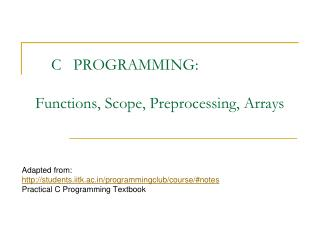# C PROGRAMMING: Functions, Scope, Preprocessing, Arrays - PowerPoint PPT PresentationDownload PresentationC PROGRAMMING: Functions, Scope, Preprocessing, Arrays

Download Presentation## C PROGRAMMING: Functions, Scope, Preprocessing, Arrays

- - - - - - - - - - - - - - - - - - - - - - - - - - - E N D - - - - - - - - - - - - - - - - - - - - - - - - - - -
##### Presentation Transcript

1. C PROGRAMMING:Functions, Scope, Preprocessing, Arrays Adapted from: http://students.iitk.ac.in/programmingclub/course/#notes Practical C Programming Textbook

2. Outline • Functions • Scope • Preprocessing • Examples

3. Some Essentials • Scope of a variable: - The areas in the code where the variable is visible. • Life of a variable: - Areas of the code where the variable exists in memory.

4. Example for( int i =0; I < 100 ; i++ ){ \\scope of i } can’t see i here. ****************************************************** int main(){ int i = 0; }// i dies after the main program ends.

5. Scope

6. Global Variables

7. Scope: An Example

8. Scope: Weirdness

9. int main() { // Declaration and Assignment int level_0 = 3; // Just declaration int answer; /* New scope begins here */ { int level_1 = 1; { int level_2 = 2; } answer = level_1 + level_2; // Error!! level_2 not visible here } /* End of scope */ /* level_1 is no more alive */ answer = level_0 + level_1; // Error! level_1 is not visible here return 0; }

10. Scope: Weirdness

11. Rule of Thumb

12. Method/Function • Definition: A block of code which performs some well defined computational task. • a method may have some input • a method may also have some output Example: int add( int , int) Can visualize as a mathematical function which takes a value and returns another value.

13. Why Methods • Huge codes are difficult to understand and debug. • Makes the code look simpler, neater and makes your task easier. • Re-usability of code. Example: Imagine a program in which we need to swap two numbers often. Life becomes easy if we could swap in a single step. printf(),scanf() are functions too. They make our work so easy.

14. Some terminologies • Function Declaration/ Prototype: int func(int , int); • Function Definition: int func ( int a, int b){ printf(“Welcome to func”); return (a + b); }

15. Some more • The value passed to a method is called its“argument” • The variable which receives the arguments is called“parameter” • Parameters are declared inside the parenthesis and we must declare the type of the parameter. • Argument may be an expression but, type (argument) = type (parameter)

16. Typical Example #include <stdio.h> void add_print(int , int); //function declaration int main(){ int a=4; int b=5; printf(“Entering ‘add_print’ function\n”); add_print(a,b); printf(“Just came from ‘add_print’ function\n”); return 0; } //function definition void add_print(int val1,int val2){ int c; printf(“The two values entered are:%d,%d \n”,val1,val2); c=val1+val2; printf(“Sum of numbers entered is:%d \n”,c); } //parameters, arguments ?????????

17. Contd. • In the above example, • ‘a’, ‘b’ are arguments to the function. • ‘val1’, ‘val2’ are the parameters of the function. • Scope and Life • ‘a’, ‘b’ • Have scope limited to the main function. • Their life is till the main exits. • ‘val1’, ‘val2’ • Have scope limited to the function block. • Their life is till the function call is over.

18. Another Example #include <stdio.h> int mult_return(int , int); //function declaration int main(){ int a=4; int b=5; int c; printf(“Entering ‘mult_return’ function\n”); c = mult_return(a+4,b*2); // sending an expression printf(“Just came from ‘mult_return’ function. The value is %d \n”, c); return 0; } //function definition int mult_return(int val1,int val2){ int c; // “c” again???? printf(“The two values entered are:%d,%d \n”,val1,val2); c=val1*val2; return c; }

19. Another Example #include <stdio.h> void swap(int , int); //function declaration int main(){ int a=4; int b=5; swap(a,b); printf(“The value of ‘a’ is %d and the value of ‘b’ is %d\n”, a,b); return 0; } // the values of a and b did not swap . //function definition void swap(int val1,int val2){ int temp; printf(“The two values entered are:%d,%d \n”,val1,val2); temp=val1; val1=val2; val2=temp; }

20. Some problems • Till now the functions took the values of the arguments from the calling function (main). • What if we need to change the values of variables in the calling function? • How do we get access to the calling function’s data? Simple!! Send the address of the variable

21. Pre Processor Directives • #include • #define • #if • #ifdef • #ifndef • #else • #elif • #endif • Used for Including Files, Conditional Compilation and Macro Definition

22. Including Files #include <stdio.h> int main (void) { printf("Hello, world!\n"); return 0; }

23. Conditional Compilation • The #if, #ifdef, #ifndef, #else, #elif and #endif directives can be used for conditional compilation. • Example 1 #define __WINDOWS__ #ifdef __WINDOWS__ #include <windows.h> #else #include <unistd.h> #endif

24. Conditional Compilation Example 2 #define __DEBUG__ #ifdef __ DEBUG __ printf("trace message"); #endif

25. Macro Definition and Expansion Object Like: #define <identifier> <replacement token list> Example: #define PI 3.14159 Function Like: #define <identifier>(<parameter list>) <replacement token list> Example: #define RADTODEG(x) ((x) * 57.29578)

26. Macro Definition and Expansion Function Like:Be careful! Examples: • #define MAC1(x) (x * 57.29578) will expand MAC1(a + b) to (a + b * 57.29578) • # define ABC(X) X*X • Replaces the occurrences of ABC(z) with z*z. • What happens to ABC(z+1)? // try out • #define MIN(a,b) ((a)>(b)?(b):(a)) What happens when called as MIN(++firstnum, secondnum) ?